Anúncio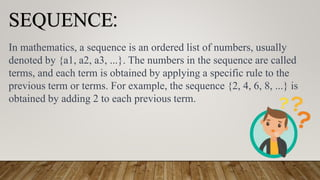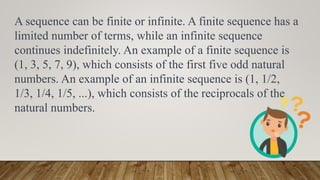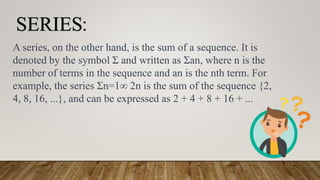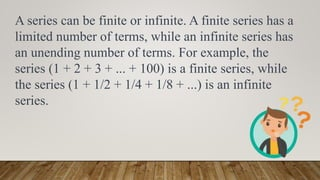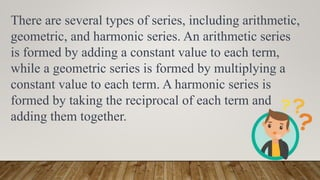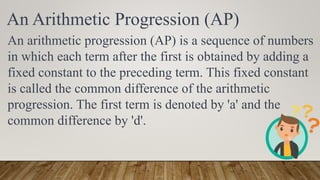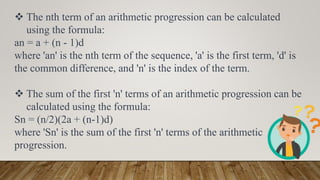1 de 9
Anúncio

### MATHS PPT 2 (2).pptx

1. BY: 1]RUTVIK WARANG ROLL NO 5
2. SEQUENCE: In mathematics, a sequence is an ordered list of numbers, usually denoted by {a1, a2, a3, ...}. The numbers in the sequence are called terms, and each term is obtained by applying a specific rule to the previous term or terms. For example, the sequence {2, 4, 6, 8, ...} is obtained by adding 2 to each previous term.
3. A sequence can be finite or infinite. A finite sequence has a limited number of terms, while an infinite sequence continues indefinitely. An example of a finite sequence is (1, 3, 5, 7, 9), which consists of the first five odd natural numbers. An example of an infinite sequence is (1, 1/2, 1/3, 1/4, 1/5, ...), which consists of the reciprocals of the natural numbers.
4. SERIES: A series, on the other hand, is the sum of a sequence. It is denoted by the symbol Σ and written as Σan, where n is the number of terms in the sequence and an is the nth term. For example, the series Σn=1∞ 2n is the sum of the sequence {2, 4, 8, 16, ...}, and can be expressed as 2 + 4 + 8 + 16 + ...
5. A series can be finite or infinite. A finite series has a limited number of terms, while an infinite series has an unending number of terms. For example, the series (1 + 2 + 3 + ... + 100) is a finite series, while the series (1 + 1/2 + 1/4 + 1/8 + ...) is an infinite series.
6. There are several types of series, including arithmetic, geometric, and harmonic series. An arithmetic series is formed by adding a constant value to each term, while a geometric series is formed by multiplying a constant value to each term. A harmonic series is formed by taking the reciprocal of each term and adding them together.
7. An Arithmetic Progression (AP) An arithmetic progression (AP) is a sequence of numbers in which each term after the first is obtained by adding a fixed constant to the preceding term. This fixed constant is called the common difference of the arithmetic progression. The first term is denoted by 'a' and the common difference by 'd'.
8.  The nth term of an arithmetic progression can be calculated using the formula: an = a + (n - 1)d where 'an' is the nth term of the sequence, 'a' is the first term, 'd' is the common difference, and 'n' is the index of the term.  The sum of the first 'n' terms of an arithmetic progression can be calculated using the formula: Sn = (n/2)(2a + (n-1)d) where 'Sn' is the sum of the first 'n' terms of the arithmetic progression.
Anúncio